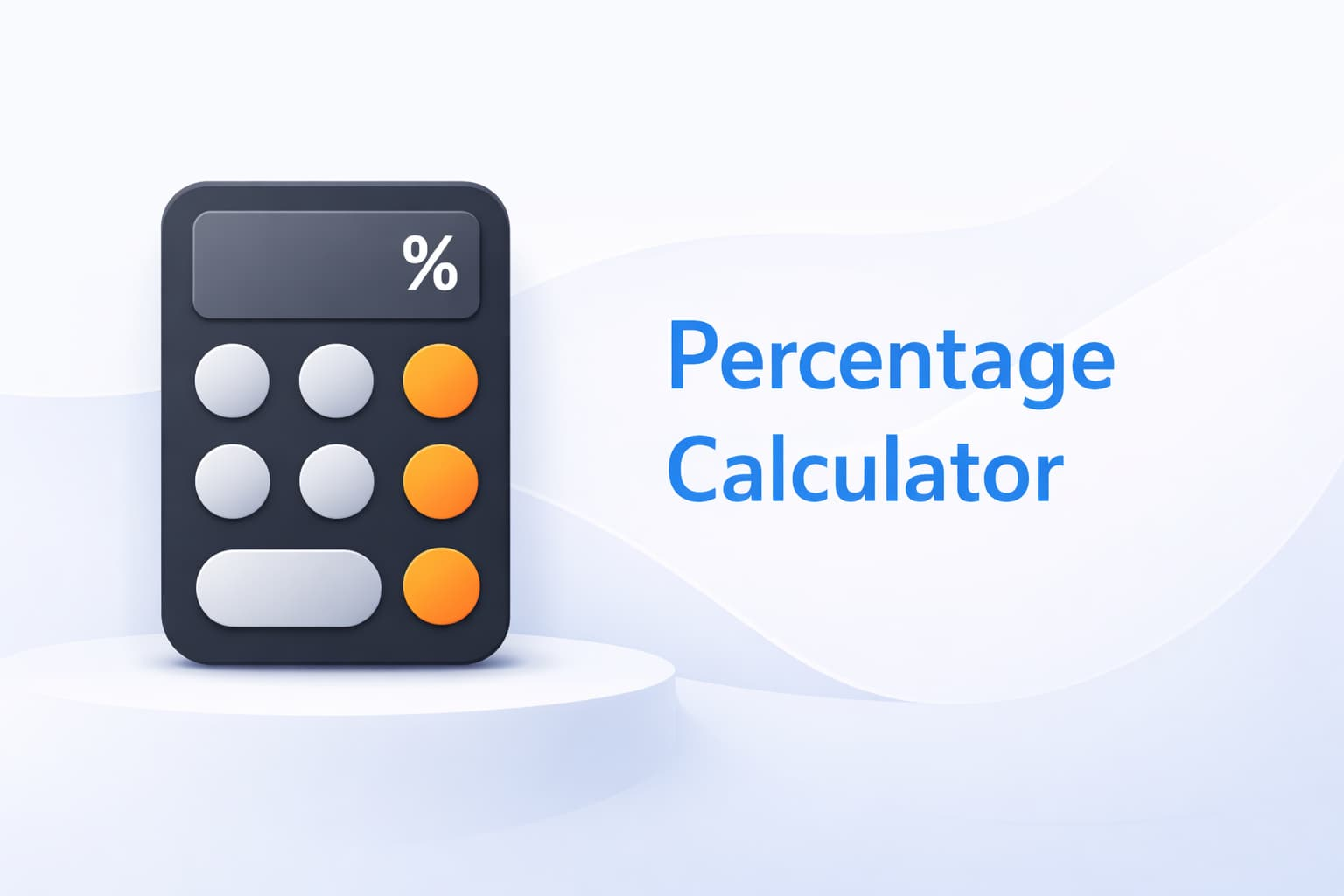# Percentage Calculator

Percentage Calculator is a free online tool to calculate percentages of a number. Please provide any two values below and click the "Calculate" button to get the third value.This percentage calculator is a tool that lets you do a simple calculation: what percent of X is Y? The tool is pretty straightforward.